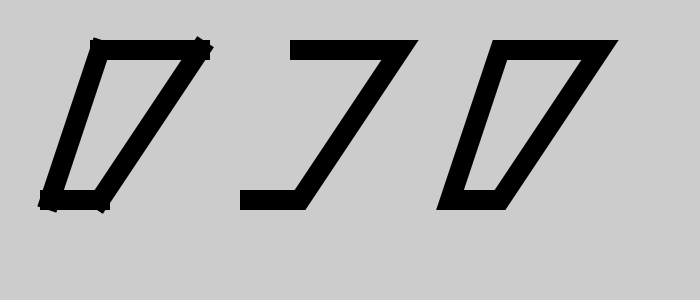# Composite paths in generativepy

Martin McBride, 2022-01-05
Tags generativepy tutorial composite path line bezier curve arc roundrect
Categories generativepy generativepy tutorialThis tutorial shows how to composite paths in generativepy. You should read through the fill and stroke tutorial first if you haven't already.

generativepy allows you to draw various basic shapes:

In this section we will see how to combine these different shapes to create new shapes. We will create multiple shape objects then join them to create a single path.

A single path created from two or more shape objects is called a composite path.

Also see the article on complex paths, which builds on the techniques discussed here.

## Composite paths with lines

We can create a composite path by combining several lines. You would rarely need to do this, because the Polygon object can do this more easily. The code is this section is just a simple example of how to create composite paths.

Here is some code that draws three versions of the same shape:

from generativepy.drawing import make_image, setup
from generativepy.color import Color
from generativepy.geometry import Line

def draw2(ctx, pixel_width, pixel_height, frame_no, frame_count):
setup(ctx, pixel_width, pixel_width, background=Color(0.8))

black = Color(0)

Line(ctx).of_start_end((100, 50), (200, 50)).stroke(black, 20)
Line(ctx).of_start_end((200, 50), (100, 200)).stroke(black, 20)
Line(ctx).of_start_end((100, 200), (50, 200)).stroke(black, 20)
Line(ctx).of_start_end((50, 200), (100, 50)).stroke(black, 20)

Line(ctx).of_end((250, 200)).extend_path().stroke(black, 20)

Line(ctx).of_end((450, 200)).extend_path(close=True).stroke(black, 20)

make_image("composite-lines.png", draw2, 700, 300)


This code is available on github in tutorial/shapes/composite-lines.py.

Here is the resulting image:The first block of code is an example of how not to do it:

    Line(ctx).of_start_end((100, 50), (200, 50)).stroke(black, 20)
Line(ctx).of_start_end((200, 50), (100, 200)).stroke(black, 20)
Line(ctx).of_start_end((100, 200), (50, 200)).stroke(black, 20)
Line(ctx).of_start_end((50, 200), (100, 50)).stroke(black, 20)


This code draws the shape on the left of the image above, by drawing four completely separate lines. While this creates the correct shape, it doesn't look right because the lines don't join correctly. That is because the lines are complete,y different objects. They just happen to overlap.

The next block of code create three Line objects as a single path. It draws the shape in the middle of image above:

    Line(ctx).of_start_end((300, 50), (400, 50)).add()
Line(ctx).of_end((250, 200)).extend_path().stroke(black, 20)


It is important to understand how this code works, so we will look at it in detail.

The first Line is created between points (300, 50) and (400, 50). It is created in the usual way. However, rather than stoking the line, we call the add() method.

add() takes the current object and store internally as the current path.

The second Line is created slightly differently. We want this line to join on to the end of the previous line, so we use of_end to supply just the new end point. The line will be drawn between the previous end point (400, 50) and the new end point (300, 200). We also call extend_path so that this new line is added to the previously stored path. We call add() again. The internal current path now contains both lines, as a single path.

The third Line follows the same pattern as the second. We use of_end() and extend_path() to create a path containing all three lines.

The third line is slightly different because we have finished the path. Rather than using add() to store the path, we use stroke() to draw it, in the usual way. Since the three lines are part of the same path, they are stroked with the correct line joins.

You will notice that the shape isn't closed. We could try closing the shape by drawing a fourth line, but that would cause a similar proble to the previous case, the first and last lines would not join correctly. Instead we must close the shape. like this:

    Line(ctx).of_start_end((500, 50), (600, 50)).add()
Line(ctx).of_end((450, 200)).extend_path(close=True).stroke(black, 20)


All we have done here is add close=True to the final extend_path. This closes the shape.

## Composite paths with arc

This code shows how to use draw wounded rectangle and "pill" shapes:

from generativepy.drawing import make_image, setup
from generativepy.color import Color
from generativepy.geometry import Circle
import math

def draw(ctx, pixel_width, pixel_height, frame_no, frame_count):
setup(ctx, pixel_width, pixel_width, background=Color(0.8))

black = Color(0)

make_image("complex-roundrect.png", draw, 700, 300)


This code is available on github in tutorial/shapes/composite-roundrect.py.

Here is the resulting image:This is the code to draw the rounded rectangle on the left:

    Circle(ctx).of_center_radius((50, 50), 10).as_arc(math.pi, math.pi*3/2).add()


This code draws the four arcs that form the corners of the rounded rectangle. Each arc has an angle of pi/2, which is a quarter of a turn.

As before, we use add() and extend_path() to create a single path from all the curves. The final extend_path() has close=True to close the shape.

You may have noticed that we have only drawn the arcs. That is deliberate. When we add an arc to a path, it will automatically draw a straight line from the previous position to the start of the arc.

The second block of code draws the pill shape on the right of the image above. This is drawn is a similar way to the rounded rectangle, but it contains two semi-circles:

    Circle(ctx).of_center_radius((350, 50), 30).as_arc(math.pi/2, math.pi*3/2).add()